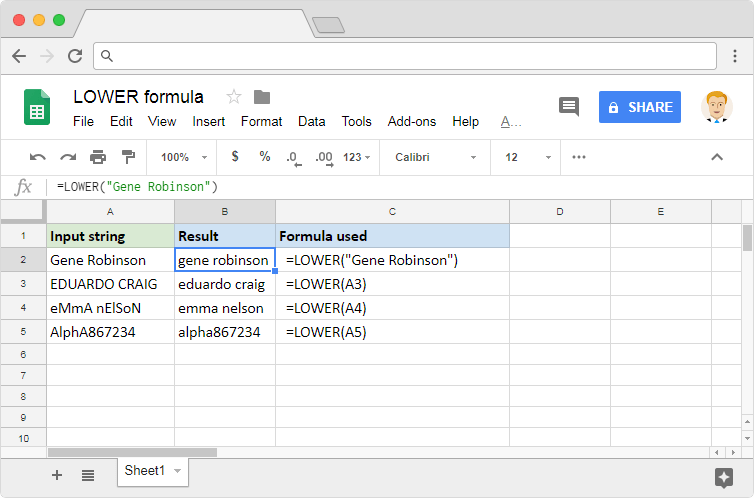# How to use the LOWER formula in Google SheetsWe know we use the spreadsheets applications generally to working with numbers. But we also have a good help in terms of a number of text-based formulas at our disposal. By making use of these, we can manipulate the strings in many ways according to our needs. The LOWER formula in Google Sheets is one such formula. It helps us to convert the text from uppercase to lowercase.

### Syntax

LOWER(text)

• text – is the input string that we need lowercase conversion for.

### Usage: LOWER formula in Google Sheets

We may have observed this is probably the simplest of syntaxes any Google Sheets formula can have. Although it is very straightforward as to what it does, let us look at a few examples to ensure we understand its behavior. Consider the snapshot below.In the first example, the formula converted the two instances of uppercase letters, G and R, are into lowercase g and r. Here the input is a direct text string, enclosed within a pair of double quotes. It may not always be feasible to type in the input strings. So, to save us from that effort, the LOWER formula also accepts references to cells that host these text values. Which is why we used cell references in the next three examples.

Regardless of the position of an uppercase character within the string, the formula converts every such instance to a lowercase character. It could be that all of the text is in uppercase (example 2), or in lowercase. Or it could also be that the characters in the input string are randomly uppercase and lowercase (example 3). It doesn’t matter how many uppercase characters there are within the input string. The result the formula always generates an output in which every alphabet is in lowercase. That is true even in the case of alphanumeric strings (example 4).